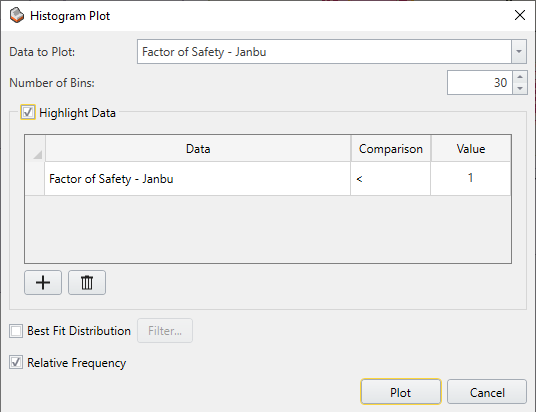The Rocscience International Conference 2021 Proceedings are now available. Read Now

# Histogram Plot

To plot histograms of results after a Probabilistic Analysis:

1. Select Histogram Plotfrom the toolbar or the Statistics > Histogram menu.
2. In the Histogram Plot dialog, select the data you would like to plot. The following data can be plotted:
• Any Random Variable that you have defined for the Probabilistic Analysis (these will appear first in the drop-down list of Data to Plot).
• The Factor of Safety for any analysis method which was used (these will be listed after the input data Random Variables).
3. The Number of Bins used to create the histogram, can be customized if desired.
4. You can also highlight data on the plot, according to user-defined criteria, by selecting the Highlight Data checkbox, and defining your criteria. See below for details.
5. You can choose Relative Frequency check to get the relative frequency of the histogram. Best fit distribution curve can also be plotted on top of the histogram.6. Select the Plot button to generate the Histogram plot.
7. Once a plot is generated, there are many different options available, if you right-click the mouse on the plot. See below for details.

## Highlight Data

To highlight any subset of data on the histogram:

1. Select the Highlight Data checkbox in the Histogram Plot dialog.
2. Select a Random Variable.
3. Select an operator (< , <= , > , >= , Range).
4. Enter the numerical value(s) defining the data you would like to see highlighted.
5. When you select the Plot button, data which fits your selection criteria, will be highlighted on the histogram in different color with legend. The highlight criterion will be listed at the top of the histogram.

EXAMPLE: plot a Histogram of an input Random Variable (e.g. a material property), and then define a Highlight Data criterion of Factor of Safety < 1. This will show the distribution of failed analyses, relative to the overall distribution of the material property.

## Histogram – Input Data Random Variables

If you are plotting a Histogram of one of your input data Random Variables, then the following information will be displayed on the plot.

• SAMPLED: the calculated statistics of the raw data which was generated by the statistical sampling (Monte Carlo or Latin Hypercube) of your input data distribution.
• INPUT: the user-defined input parameters of the distribution, that you defined for the Random Variable in the Slide Model program. In general, the parameters of the input distribution (mean, standard deviation, minimum and maximum), will NOT be exactly equal to the parameters calculated for the raw (sampled) data.

NOTE: this information will only be listed if the Input Distribution is displayed on the Histogram. The display can be toggled on or off by selecting the Input Distribution option from the right-click menu.

## Histogram – Factor of Safety

If you are plotting a Histogram of Safety Factor, then the following information will be displayed on the plot.

• SAMPLED: the calculated statistics of the raw safety factor data generated by the Probabilistic Analysis.
• PROBABILITY OF FAILURE: the Probability of Failure (PF = ###) for the selected Analysis Method.
• RELIABILITY INDEX: the Reliability Index (RI = ###) for the selected Analysis Method.
• FIT: the statistics of the BEST FIT distribution, to the raw data. The type of distribution, and the parameters of the distribution, which best fits the actual distribution of the raw safety factor data, is listed. In most cases, the statistics of the FIT distribution, will be identical to the statistics of the raw data.

NOTE: this information will only be listed if the Best Fit Distribution is displayed on the Histogram. The display can be toggled on or off by selecting the Best Fit Distribution option from the right-click menu.

## Right Click Options

Many different options are available if you right-click on a Histogram. For example:

• Select the Change Plot Data option, and the Histogram Plot dialog will appear, allowing you to change the data viewed on the plot.
• Select the Chart Properties option to customize the appearance of the histogram.
• Select Input Distribution to toggle the display of the actual distribution that you defined for the Random Variable (only applicable for input data Random Variables)
• Select Best Fit Distribution to toggle the display of the Best Fit distribution to the histogram data (only applicable for Factor of Safety histograms)
• Select Highlight Data Only to see only the highlighted data on the graph.
• Plot in Excel: you can either right click a chart or click the 'export to excel' button in the toolbar. An Excel application will open and the data will be exported in columns, and a chart object duplicating what was in the Rocscience program will also appear.
• Copy Data to Clipboard: allows you to copy and paste the data to other spreadsheet or word file.

Experiment with the different options available in the right-click menu.